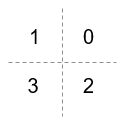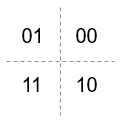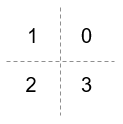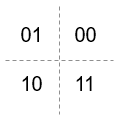# comm.QPSKDemodulator

(To be removed) Demodulate using QPSK method

comm.QPSKDemodulator will be removed in a future release. Use `pskdemod` instead. For information on updating your code, see Version History.

## Description

The `comm.QPSKDemodulator` object demodulates a signal that was modulated using the quadrature phase shift keying (QPSK) method. The input is a baseband representation of the modulated signal.

To demodulate a signal that was modulated using the QPSK method:

1. Create the `comm.QPSKDemodulator` object and set its properties.

2. Call the object with arguments, as if it were a function.

## Creation

### Syntax

``qpskdemod = comm.QPSKDemodulator``
``qpskdemod = comm.QPSKDemodulator(Name=Value)``
``qpskdemod = comm.QPSKDemodulator(phase=Name,Value)``

### Description

``qpskdemod = comm.QPSKDemodulator` creates a System object™ to demodulate input QPSK signals.`

example

````qpskdemod = comm.QPSKDemodulator(Name=Value)` sets properties using one or more name-value arguments. For example, `DecisionMethod="Hard decision"` specifies demodulation using the hard-decision method.```
````qpskdemod = comm.QPSKDemodulator(phase=Name,Value)` sets the `PhaseOffset` property to `phase`, and optional name-value arguments. Specify `phase` in radians.```

## Properties

expand all

Unless otherwise indicated, properties are nontunable, which means you cannot change their values after calling the object. Objects lock when you call them, and the `release` function unlocks them.

If a property is tunable, you can change its value at any time.

Phase of the zeroth point in the constellation in radians, specified as a scalar.

Example: `PhaseOffset=0` aligns the QPSK signal constellation points on the axes {(1,0), (0,j), (-1,0), (0,-j)}.

Data Types: `double`

Option to output data as bits, specified as a logical `0` (`false`) or `1` (`true`).

• Set this property to `false` to output symbols as integer values in the range [`0`, `3`] with length equal to the input data vector length.

• Set this property to `true` to output a column vector of bit values with length equal to twice the input data vector length.

Data Types: `logical`

Symbol encoding mapping of constellation bits, specified as `'Gray'` or `'Binary'`.

SettingConstellation Mapping for IntegersConstellation Mapping for BitsComment

`Gray`Map symbols using Gray-coded ordering.

`Binary`Map symbols using natural binary-coded ordering. The signal constellation maps to the complex value ```ej(PhaseOffset + (2πm/4))```, where `m` is an integer in the range [0, 3].

Demodulation decision method, specified as `'Hard decision'`, `'Log-likelihood ratio'`, or ```'Approximate log-likelihood ratio'```. When you set the `BitOutput` property to `false`, the object always performs hard-decision demodulation.

#### Dependencies

To enable this property, set the `BitOutput` property to `true`.

Source of noise variance, specified as `'Property'` or `'Input port'`.

#### Dependencies

To enable this property, set the `BitOutput` property to `true` and the `DecisionMethod` property to `'Log-likelihood ratio'` or ```'Approximate log-likelihood ratio'```.

Noise variance, specified as a positive scalar.

Tunable: Yes

#### Tips

The exact LLR algorithm computes exponentials using finite precision arithmetic. For computations involving very large positive or negative magnitudes, the exact LLR algorithm yields:

• `Inf` or `-Inf` if the noise variance is a very large value

• `NaN` if the noise variance and signal power are both very small values

The approximate LLR algorithm does not compute exponentials. You can avoid `Inf`, `-Inf`, and `NaN` results by using the approximate LLR algorithm.

#### Dependencies

To enable this property, set the `BitOutput` property to `true`, the `DecisionMethod` property to `'Log-likelihood ratio'` or ```'Approximate log-likelihood ratio'```, and the `VarianceSource` property to `'Property'`.

Data Types: `double`

Data type of the output, specified as `'Full precision'`, `'Smallest unsigned integer'`, `'double'`, `'single'`, `'int8'`, `'uint8'`, `'int16'`, `'uint16'`, `'int32'`, or `'uint32'`,`'logical'`.

• When the input data type is single or double precision and you set the `BitOutput` property to `true`, the `DecisionMethod` property to `'Hard decision'`, and the `OutputDataType` property to `'Full precision'`, the output has the same data type as that of the input.

• When the input data is of a fixed-point type, the output data type behaves as if you had set the `OutputDataType` property to `'Smallest unsigned integer'`.

• When you set `BitOutput` to `true` and the `DecisionMethod` property to ```'Hard Decision'```, then `'logical'` data type is a valid option.

• When you set the `BitOutput` property to `true` and the `DecisionMethod` property to ```'Log-likelihood ratio'``` or `'Approximate log-likelihood ratio'`, the output data type is the same as that of the input and the input data type must be single or double precision.

#### Dependencies

To enable this property, set the `BitOutput` property to `false` or set the `BitOutput` property to `true` and the `DecisionMethod` property to `'Hard decision'`.

Fixed-Point Properties

Data type of the derotate factor, specified as ```'Same word length as input'``` or `'Custom'`. The object uses the derotate factor in the computations only when the input signal is a fixed-point type and the `PhaseOffset` property has a value that is not an even multiple of π/4.

#### Dependencies

To enable this property, set the `BitOutput` property to `false` or set the `BitOutput` property to `true` and the `DecisionMethod` property to `'Hard decision'`.

Fixed-point data type of the derotate factor, specified as an unscaled `numerictype` (Fixed-Point Designer) object with a `Signedness` of `Auto`.

#### Dependencies

To enable this property, set the `DerotateFactorDataType` property to `'Custom'`.

Data Types: `numerictype object`

## Usage

### Syntax

``y = qpskdemod(x)``
``y = qpskdemod(x,var)``

### Description

example

````y = qpskdemod(x)` applies QPSK demodulation to the input signal and returns the demodulated signal.```
````y = qpskdemod(x,var)` uses soft decision demodulation and noise variance `var`. This syntax applies when you set the `BitOutput` property to `true`, the `DecisionMethod` property to `'Approximate log-likelihood ratio'` or ```'Log-likelihood ratio'```, and the `VarianceSource` property to `'Input port'`.```

### Input Arguments

expand all

QPSK-modulated signal, specified as a scalar or column vector.

#### Dependencies

The object accepts inputs with a signed integer data type or signed fixed point (`sfi` (Fixed-Point Designer)) objects when you set the `BitOutput` property to `false` or you set the `DecisionMethod` property to `'Hard decision'` and the `BitOutput` property to `true`.

Data Types: `double` | `single` | `int` | `fi`

Noise variance, specified as a scalar.

#### Dependencies

To enable this argument, set the `VarianceSource` property to `'Input port'`, the `BitOutput` property to `true`, and the `DecisionMethod` property to `'Approximate log-likelihood ratio'` or ```'Log-likelihood ratio'```.

Data Types: `single` | `double`

### Output Arguments

expand all

Output signal, returned as a scalar or column vector. To specify whether the object outputs values as integers or bits, use the `BitOutput` property. To specify the output data type, use the `OutputDataType` property.

## Object Functions

To use an object function, specify the System object as the first input argument. For example, to release system resources of a System object named `obj`, use this syntax:

`release(obj)`

expand all

 `constellation` Calculate or plot ideal signal constellation
 `step` Run System object algorithm `release` Release resources and allow changes to System object property values and input characteristics `reset` Reset internal states of System object

## Examples

collapse all

Estimate the bit error rate of a QPSK modulated signal filtered through an AWGN channel.

Create a QPSK modulator and demodulator pair that operate on bits.

```qpskModulator = comm.QPSKModulator('BitInput',true); qpskDemodulator = comm.QPSKDemodulator('BitOutput',true);```

Create an AWGN channel object and an error rate counter.

```channel = comm.AWGNChannel('EbNo',4,'BitsPerSymbol',2); errorRate = comm.ErrorRate;```

Generate random binary data and apply QPSK modulation.

```data = randi([0 1],1000,1); txSig = qpskModulator(data);```

Pass the signal through the AWGN channel and demodulate it.

```rxSig = channel(txSig); rxData = qpskDemodulator(rxSig);```

Calculate the error statistics. Display the BER.

```errorStats = errorRate(data,rxData); errorStats(1)```
```ans = 0.0100 ```

expand all

## Version History

Introduced in R2012a

expand all